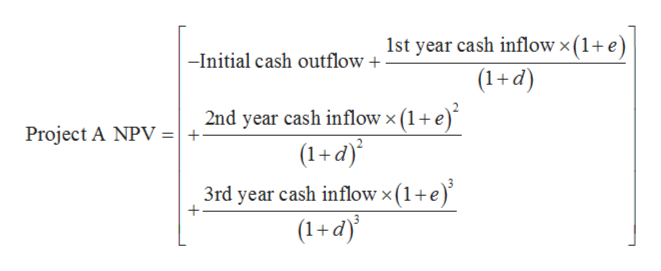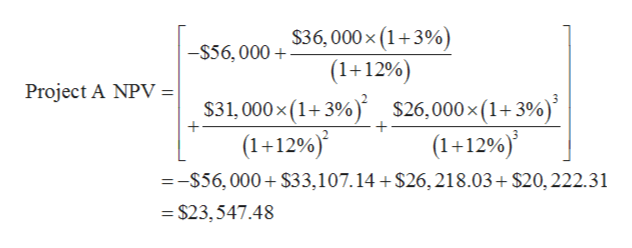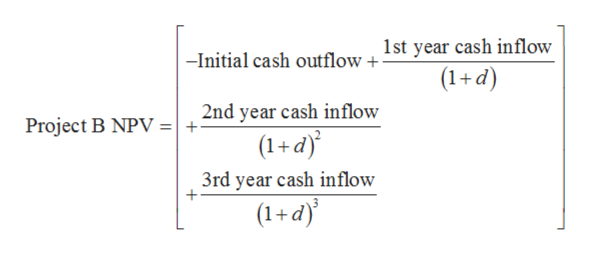# Consider the following cash flows on two mutually exclusive projects:  YearProject AProject B0    –\$56,000    –\$71,000  1   36,000   35,000   2   31,000   44,000  3    26,000   47,000     The cash flows of Project A are expressed in real terms, whereas those of Project B are expressed in nominal terms. The appropriate nominal discount rate is 12 percent and the inflation rate is 3 percent.  Calculate the NPV for each project. (Do not round intermediate calculations and round your answers to 2 decimal places, e.g., 32.16.) NPV FOR PROJECT A:NPV FOR PROJECT B:

Question
14 views
 Consider the following cash flows on two mutually exclusive projects:
 Year Project A Project B 0 –\$ 56,000 –\$ 71,000 1 36,000 35,000 2 31,000 44,000 3 26,000 47,000

 The cash flows of Project A are expressed in real terms, whereas those of Project B are expressed in nominal terms. The appropriate nominal discount rate is 12 percent and the inflation rate is 3 percent.

 Calculate the NPV for each project. (Do not round intermediate calculations and round your answers to 2 decimal places, e.g., 32.16.)

NPV FOR PROJECT A:
NPV FOR PROJECT B:

check_circle

Step 1

Cash flows given for project A are in real terms, so real discount rate should be used to calculate their present value. This can be done by considering inflation in the calculation.

The net present value for project A can be calculated with the help of below expression:help_outlineImage Transcriptionclose1st year cash inflow x (1+ e) (1+d) -Initial cash outflow + 2nd year cash inflowx (1+e) (1+d) 3rd year cash inflow x (1 + e) (1+d)' Project A NPV + fullscreen
Step 2

The net present value for project A is calculated below:help_outlineImage Transcriptionclose\$36, 000 x (1+3%) (1+12%) -\$56,000 Project A NPV | \$31,000x (1+3%) \$26,000x(1+3%) (1+12%) =-S56,000 \$33,107. 14+ \$26,218.03+ \$20,222.31 (1+1296) =\$23,547.48 fullscreen
Step 3

Cash flows given for project B are in nominal terms, so given nominal discount rate should be used to calculate their...help_outlineImage Transcriptionclose1st year cash inflow -Initial cash outflow + (1+d) 2nd year cash inflow + (1+d) Project B NPV 3rd year cash inflow (1+d) fullscreen

### Want to see the full answer?

See Solution

#### Want to see this answer and more?

Solutions are written by subject experts who are available 24/7. Questions are typically answered within 1 hour.*

See Solution
*Response times may vary by subject and question.
Tagged in